# Tree Traversal | Binary Tree Traversal

## Binary Tree-

Before you go through this article, make sure that you gone through the previous article on Binary Trees.

We have discussed-

• Binary tree is a special tree data structure.
• In a binary tree, each node can have at most 2 children.

## Tree Traversal-

 Tree Traversal refers to the process of visiting each node in a tree data structure exactly once.

Various tree traversal techniques are-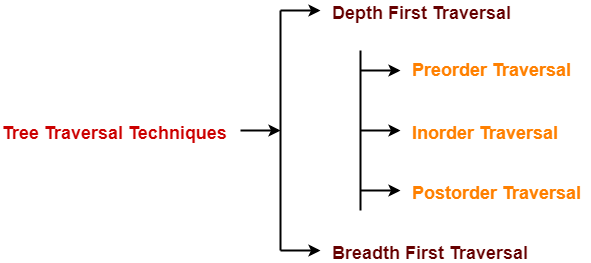## Depth First Traversal-

Following three traversal techniques fall under Depth First Traversal-

1. Preorder Traversal
2. Inorder Traversal
3. Postorder Traversal

## Algorithm-

1. Visit the root
2. Traverse the left sub tree i.e. call Preorder (left sub tree)
3. Traverse the right sub tree i.e. call Preorder (right sub tree)

Root  Left  Right

## Example-

Consider the following example-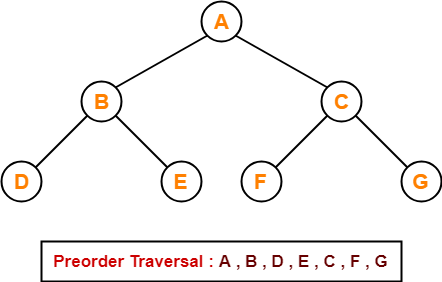## Preorder Traversal Shortcut

Traverse the entire tree starting from the root node keeping yourself to the left.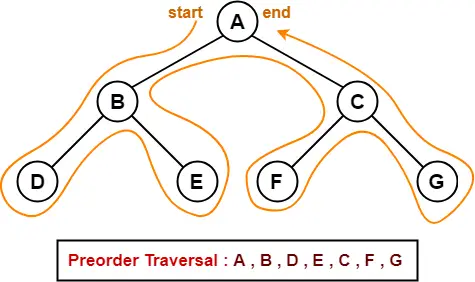## Applications-

• Preorder traversal is used to get prefix expression of an expression tree.
• Preorder traversal is used to create a copy of the tree.

## Algorithm-

1. Traverse the left sub tree i.e. call Inorder (left sub tree)
2. Visit the root
3. Traverse the right sub tree i.e. call Inorder (right sub tree)

Left  Root  Right

## Example-

Consider the following example-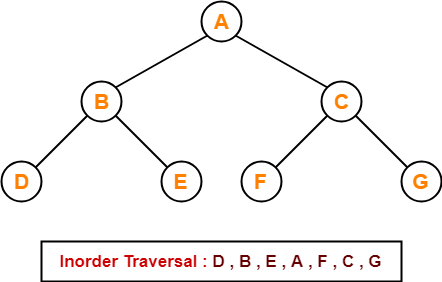## Inorder Traversal Shortcut

Keep a plane mirror horizontally at the bottom of the tree and take the projection of all the nodes.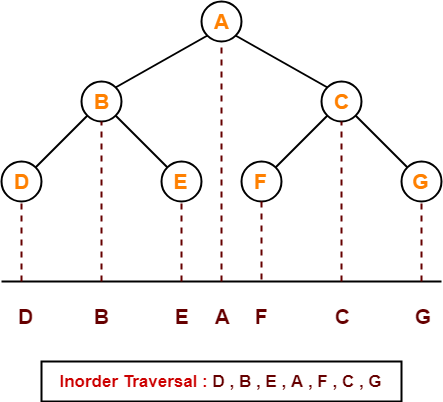## Application-

• Inorder traversal is used to get infix expression of an expression tree.

## Algorithm-

1. Traverse the left sub tree i.e. call Postorder (left sub tree)
2. Traverse the right sub tree i.e. call Postorder (right sub tree)
3. Visit the root

Left  Right  Root

## Example-

Consider the following example-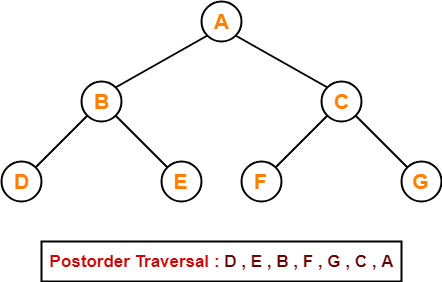## Postorder Traversal Shortcut

Pluck all the leftmost leaf nodes one by one.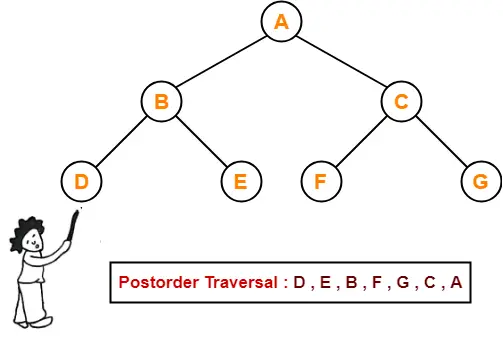## Applications-

• Postorder traversal is used to get postfix expression of an expression tree.
• Postorder traversal is used to delete the tree.
• This is because it deletes the children first and then it deletes the parent.

• Breadth First Traversal of a tree prints all the nodes of a tree level by level.
• Breadth First Traversal is also called as Level Order Traversal.

## Example-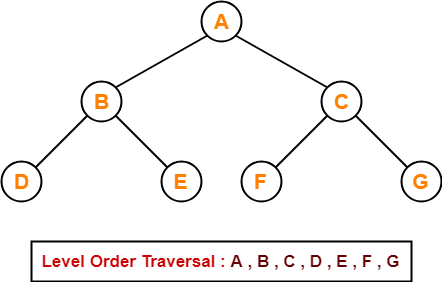## Application-

• Level order traversal is used to print the data in the same order as stored in the array representation of a complete binary tree.

To gain better understanding about Tree Traversal,

Watch this Video Lecture

## Problem-01:

If the binary tree in figure is traversed in inorder, then the order in which the nodes will be visited is ____?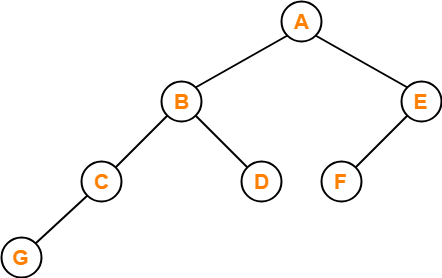## Solution-

The inorder traversal will be performed as-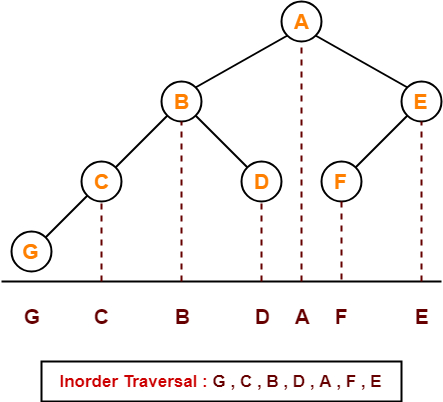## Problem-02:

Which of the following sequences denotes the postorder traversal sequence of the tree shown in figure?1. FEGCBDBA
2. GCBDAFE
3. GCDBFEA
4. FDEGCBA

## Solution-

Perform the postorder traversal by plucking all the leftmost leaf nodes one by one.

Then,

Postorder Traversal :  G , C , D , B , F , E , A

Thus, Option (C) is correct.

## Problem-03:

Let LASTPOST, LASTIN, LASTPRE denote the last vertex visited in a postorder, inorder and preorder traversal respectively of a complete binary tree. Which of the following is always true?

1. LASTIN = LASTPOST
2. LASTIN = LASTPRE
3. LASTPRE = LASTPOST
4. None of these

## Solution-

Consider the following complete binary tree-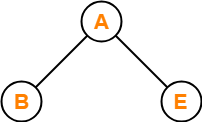Preorder Traversal  : B , A , E

Inorder Traversal     : B , A , E

Postorder Traversal : B , E , A

Clearly, LASTIN = LASTPRE.

Thus, Option (B) is correct.

## Problem-04:

Which of the following binary trees has its inorder and preorder traversals as BCAD and ABCD respectively-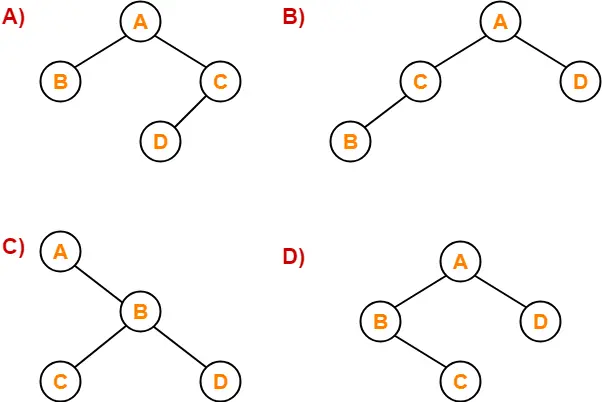## Solution-

Option (D) is correct.

To watch video solutions and practice more problems,

Watch this Video LectureNext Article- Binary Search Trees

Get more notes and other study material of Data Structures.

Watch video lectures by visiting our YouTube channel LearnVidFun.

SummaryArticle Name
Tree Traversal | Binary Tree Traversal
Description
Tree Traversal is the process of visiting each node exactly once. Binary Tree Traversal Techniques- Preorder Traversal, Inorder Traversal, Postorder Traversal, Level Order Traversal.
Author
Publisher Name
Gate Vidyalay
Publisher Logo